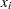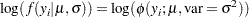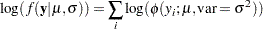Modeling Joint Likelihood

PROC MCMC assumes that the input observations are independent and that the joint log likelihood is the sum of individual log-likelihood functions. You specify the log likelihood of one observation in the MODEL statement. PROC MCMC evaluates that function for each observation in the data set and cumulatively sums them up. If observations are not independent of each other, this summation produces the incorrect log likelihood.

There are two ways to model dependent data. You can either use the DATA step LAG function or use the PROC option JOINTMODEL. The LAG function returns values of a variable from a queue. As PROC MCMC steps through the data set, the LAG function queues each data set variable, and you have access to the current value as well as to all previous values of any variable. If the log likelihood for observationdepends only on observationstoin the data set, you can use this SAS function to construct the log-likelihood function for each observation. Note that the LAG function enables you to access observations from different rows, but the log-likelihood function in the MODEL statement must be generic enough that it applies to all observations. See Time Independent Cox Model and Time Dependent Cox Model for how to use this LAG function.

A second option is to create arrays, store all relevant variables in the arrays, and construct the joint log likelihood for the entire data set instead of for each observation. Following is a simple example that illustrates the usage of this option. For a more realistic example that models dependent data, see Time Independent Cox Model and Time Dependent Cox Model.

/* allocate the sample size. */
data exi;
call streaminit(17);
do ind = 1 to 100;
y = rand("normal", 2.3, 1);
output;
end;
run;

The log-likelihood function for each observation is as follows:The joint log-likelihood function is as follows:The following statements fit a simple model with an unknown mean (mu) in PROC MCMC, with the variance in the likelihood assumed known. The MODEL statement indicates a normal likelihood for each observation y.

proc mcmc data=exi seed=7 outpost=p1;
parm mu;
prior mu ~ normal(0, sd=10);
model y ~ normal(mu, sd=1);
run;

The following statements show how you can specify the log-likelihood function for the entire data set:

data a;
run;

proc mcmc data=a seed=7 outpost=p2 jointmodel;
array data / nosymbols;
begincnst;
n = dim(data, 1);
endcnst;

parm mu;
prior mu ~ normal(0, sd=10);
ll = 0;
do i = 1 to n;
ll = ll + lpdfnorm(data[i], mu, 1);
end;
model general(ll);
run;

The JOINTMODEL option indicates that the function used in the MODEL statement calculates the log likelihood for the entire data set, rather than just for one observation. Given this option, the procedure no longer steps through the input data during the simulation. Consequently, you can no longer use any data set variables to construct the log-likelihood function. Instead, you store the data set in arrays and use arrays instead of data set variables to calculate the log likelihood.

The ARRAY statement allocates a temporary array (data). The READ_ARRAY function selects the y variable from the exi data set and stores it in the data array. See the section READ_ARRAY Function. In the programming statements, you use a DO loop to construct the joint log likelihood. The expression ll in the GENERAL function now takes the value of the joint log likelihood for all data.

You can run the following statements to see that two PROC MCMC runs produce identical results.

proc compare data=p1 compare=p2;
var mu;
run;